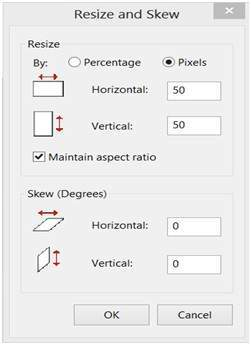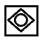# Pixel Resolution

Before we define pixel resolution, it is necessary to define a pixel.

## Pixel

We have already defined a pixel in our tutorial of concept of pixel, in which we define a pixel as the smallest element of an image. We also defined that a pixel can store a value proportional to the light intensity at that particular location.

Now since we have defined a pixel, we are going to define what is resolution.

## Resolution

The resolution can be defined in many ways. Such as pixel resolution, spatial resolution, temporal resolution, spectral resolution. Out of which we are going to discuss pixel resolution.

You have probably seen that in your own computer settings, you have monitor resolution of 800 x 600, 640 x 480 e.t.c

In pixel resolution, the term resolution refers to the total number of count of pixels in an digital image. For example. If an image has M rows and N columns, then its resolution can be defined as M X N.

If we define resolution as the total number of pixels, then pixel resolution can be defined with set of two numbers. The first number the width of the picture, or the pixels across columns, and the second number is height of the picture, or the pixels across its width.

We can say that the higher is the pixel resolution, the higher is the quality of the image.

We can define pixel resolution of an image as 4500 X 5500.

## Megapixels

We can calculate mega pixels of a camera using pixel resolution.

Column pixels (width ) X row pixels ( height ) / 1 Million.

The size of an image can be defined by its pixel resolution.

Size = pixel resolution X bpp ( bits per pixel )

## Calculating the mega pixels of the camera

Lets say we have an image of dimension: 2500 X 3192.

Its pixel resolution = 2500 * 3192 = 7982350 bytes.

Dividing it by 1 million = 7.9 = 8 mega pixel (approximately).

## Aspect ratio

Another important concept with the pixel resolution is aspect ratio.

Aspect ratio is the ratio between width of an image and the height of an image. It is commonly explained as two numbers separated by a colon (8:9). This ratio differs in different images, and in different screens. The common aspect ratios are:

1.33:1, 1.37:1, 1.43:1, 1.50:1, 1.56:1, 1.66:1, 1.75:1, 1.78:1, 1.85:1, 2.00:1, e.t.c

Aspect ratio maintains a balance between the appearance of an image on the screen, means it maintains a ratio between horizontal and vertical pixels. It does not let the image to get distorted when aspect ratio is increased.

### For example

This is a sample image, which has 100 rows and 100 columns. If we wish to make is smaller, and the condition is that the quality remains the same or in other way the image does not get distorted, here how it happens.

### Original imageChanging the rows and columns by maintain the aspect ratio in MS Paint.### ResultSmaller image, but with same balance.

You have probably seen aspect ratios in the video players, where you can adjust the video according to your screen resolution.

Finding the dimensions of the image from aspect ratio:

Aspect ratio tells us many things. With the aspect ratio, you can calculate the dimensions of the image along with the size of the image.

## For example

If you are given an image with aspect ratio of 6:2 of an image of pixel resolution of 480000 pixels given the image is an gray scale image.

And you are asked to calculate two things.

• Resolve pixel resolution to calculate the dimensions of image
• Calculate the size of the image

### Given:

Aspect ratio: c:r = 6:2

Pixel resolution: c * r = 480000

Bits per pixel: grayscale image = 8bpp

### Find:

Number of rows = ?

Number of cols = ?

### Solving first part:### Solving 2nd part:

Size = rows * cols * bpp

Size of image in bits = 400 * 1200 * 8 = 3840000 bits

Size of image in bytes = 480000 bytes

Size of image in kilo bytes = 48 kb (approx).